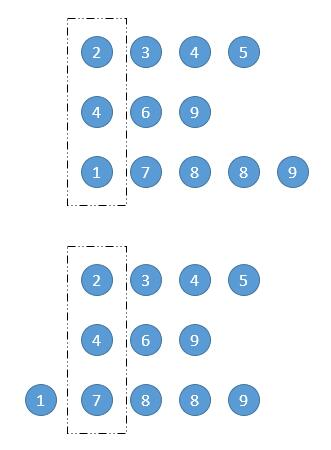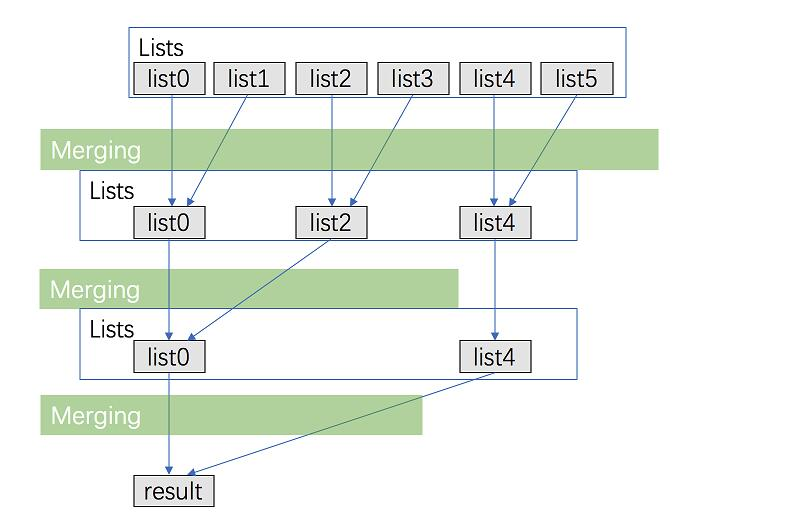# Merge K Sorted Lists

Let’s continue…

Problem Statement:

Merge K Sorted Lists

Given a linked list, remove the n-th node from the end of list and return its head.

Example:

```Given linked list: 1->2->3->4->5, and n = 2.

After removing```

Merge k sorted linked lists and return it as one sorted list. Analyze and describe its complexity.

Example:

```Input:
[
1->4->5,
1->3->4,
2->6
]
Output: 1->1->2->3->4->4->5->6
```

Solution One: Brute Force

We iterate all the numbers of all lists, and store them in an array.  After that, we sort the array and transfer the result to a new list. After that, output the final result.

Time Complexity: O(n*log(n)) We construct the array, which takes O(n) time, and sorting the array takes O(n*log(n)) time. n is the number of total nodes in all lists.

Space Complexity: O(n)

This file contains bidirectional Unicode text that may be interpreted or compiled differently than what appears below. To review, open the file in an editor that reveals hidden Unicode characters.

 /** * Definition for singly-linked list. * struct ListNode { * int val; * ListNode *next; * ListNode(int x) : val(x), next(NULL) {} * }; */ class Solution { public: ListNode* mergeKLists(vector& lists) { vector myVector; int len_l = lists.size(); for(int i = 0; i < len_l; i++){ ListNode* temp = lists[i]; while(temp){ myVector.push_back(temp->val); temp = temp->next; } } sort(myVector.begin(), myVector.end()); ListNode* head = new ListNode(0); ListNode* p_NewList = head; len_l = myVector.size(); for(int i = 0; i < len_l; i++){ ListNode* temp = new ListNode(myVector[i]); p_NewList->next = temp; p_NewList = p_NewList->next; } return head->next; } };

Solution Two: Compare Lists by Columns

The general idea is like the picture demonstrated below. We compare all the lists in one column. We first find the smallest number of this column and make it our head node,  then we move this list forward, and compare the new column again, until we find the second smallest element, then we connect our head node to this node. After that, we move forward the second list and continue this progress until all lists are empty.

Note here we do a tiny trick that we always connect the current smallest node to the previous smallest node, so we can make the space complexity O(1).Time Complexity: O(K*N), N is the length of the longest sub list. K is the number of the lists.

Space Complexity: O(1)

This file contains bidirectional Unicode text that may be interpreted or compiled differently than what appears below. To review, open the file in an editor that reveals hidden Unicode characters.

 /** * Definition for singly-linked list. * struct ListNode { * int val; * ListNode *next; * ListNode(int x) : val(x), next(NULL) {} * }; */ class Solution { public: ListNode* mergeKLists(vector& lists) { int len_l = lists.size(); ListNode head(0); ListNode *h = &head; while(true){ bool isBreak = true; int minVal = INT_MAX; int min_index = 0; for(int i = 0; i < len_l; i++) { if(lists[i] != NULL){ isBreak = false; if(lists[i]->val <= minVal) { min_index = i; minVal = lists[i]->val; } } } if(isBreak) break; h->next = lists[min_index]; h = h->next; lists[min_index] = lists[min_index]->next; } return head.next; } };

Solution Three: Priority Queue

This idea is based on the Solution Two. In Solution Two, we can see that, each time when we want to find the current smallest element, we need to iterate all the lists, which takes O(K) time. When we find this element, we connect it with previous found nodes, we need O(1) time. Can we do better here?

We can utilize the properties of priority queue to accelerate the searching process. For the first time, we just add all the first elements to our priority queue. We maintain our priority and make sure that the smallest element should always on top (first pop out). If we want the smallest number, we can easily pop the top element out of the priority queue. This operation will take O(log(k)) time because we need to balance the remaining elements and make sure the second smallest on top of the priority queue. The same thing as insert, it takes O(log (k)) time.

The rest of the idea is the same. If one list contains the current smallest element, we pop out that node, and move forward that list one Node ahead.

Time Complexity: O(max(k, n* log(k))), k is the number of sub lists

Space Complexity: O(k)

This file contains bidirectional Unicode text that may be interpreted or compiled differently than what appears below. To review, open the file in an editor that reveals hidden Unicode characters.

 /** * Definition for singly-linked list. * struct ListNode { * int val; * ListNode *next; * ListNode(int x) : val(x), next(NULL) {} * }; */ class Solution { public: ListNode* mergeKLists(vector& lists) { int len_l = lists.size(); ListNode head(0); ListNode *h = &head; auto comp = [](ListNode* l1, ListNode* l2){ return l1->val> l2->val; }; priority_queue, decltype(comp)> q(comp); for(int i = 0; i < len_l; i++){ if(lists[i]) q.push(lists[i]); } while(q.empty() == false){ h->next = q.top(); q.pop(); h = h->next; if(h->next != NULL) q.push(h->next); } return head.next; } };

Solution Four: Combine two

Look at the picture below. Note we are going to merge two lists at one time. We keep dividing the remaining lists into different two pairs, and keep merging them until we are done. For example, in the first iteration, we merge list0 and list1; list2 and list3; list4 and list5. The second iteration, we merge list0 and list2; list4….

We did this trick to reduce total times of merging. The merging process will consumes O(n’), n’ is the average number of all lists. We will do the merging process log(k) times instead of k, so the time complexity is O(n’*log(k)).

Time Complexity: O(n’*log(k))

Space Complexity: (1)This file contains bidirectional Unicode text that may be interpreted or compiled differently than what appears below. To review, open the file in an editor that reveals hidden Unicode characters.